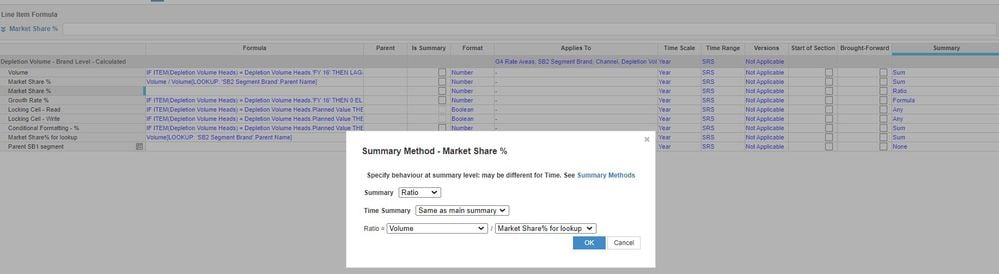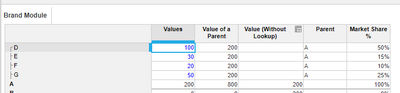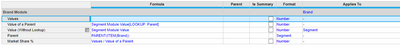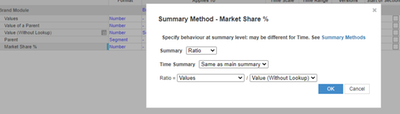# Percentage CalculationHi All,

I am facing an issue in the calculation of percentages at summary level.I have a module where I have a percentage calculation field where the percentages are calculated and are imported to another line item from there in the same module. As the second line item displays the option of user inputting the value under one of the headers but displays the calculated imported values under the other headers.

So the summary option of the 2nd line item where data is imported and is input by the user is kept as ratio to display the correct values at summary. This is because the summary level calculations of percentages is not imported.However the summary level calculation is not coming out to be correct the formula used in ratio summary is of (volume of each child(brand)/ Volume of its parent(segment)).

For all the particular brands the summary calculation is correct at an all country level, at all channel level. But the percentage calculation of the segments i.e. of the parents is coming incorrect as the denominator calculation tends to add up for all the respective brand i.e. diving each segment value by 5 times of its sum if 5 brands lie under it.Therefore the resultant value is never coming to be 100%.

I have attached the images for your reference. Requesting your help on this as this is very crucial.FYI: The first Market share% is the line item from which the values are imported to market share% line item which has no formula at the most granular level and are coming correct. The market share% for lookup is the denominator in the ratio which is coming to be incorrect at SB1 segment level (which is the parent of SB2 Segment Brand child list) however the calculations for SB2 Segment brand are coming to be correct at any of the summary levels.

Thanks

Tagged:

•That can be because your Summary is kept at SUM for both Num and Denom. Can you just create a simple calcs in excel or in anaplan and show what is  incorrect figure and what is expected outcome so that it will help us to recreate and get you close towards the solution.

•if you need different summary calculations for the different dimensions, you are going to have to detect the dim&level inside the formula and use the Formula summary type.

For example: IF low level then data else if SummaryOfThisDIm then this formula else that formula

it's a bit complex but it does work
•Hi @Misbah

The calculations are suppose to be as follows:

 SB1 Values A 200 B 300 C 400
 SB2 values Value of A market share% child of A D 50 200 25% child of A E 50 200 25% child of A F 50 200 25% parent A 200 200 100%

However in Anaplan when I am performing this calculation, the percentages are being calculated correctly for all the child list items but not for the parent SB1 market share%. The reason being is that numerator calculations are correct but however when the denominator calculation is concerned for the parent it is taking sum of all parent values of the brands i.e dividing 200 by 600 as shown below:

 SB2 values Value of A market share% in Anaplan child of A D 50 200 25% child of A E 50 200 25% child of A F 50 200 25% parent A 200 600 33%

I want to restrict the summary of denominator to formula instead of sum but I am not being able to due to the presence of lookup.

I'll be really thankful to you if you could help me resolve this or provide me an alternative solution for the same.

•Hi

@nathan_rudman,

Can you please help me understand on how to apply formula to summary. I am not being able to execute it.

•you just need to select 'Formula' as you summary method

•How do I do that my denominator has lookup in the formula
•See if this helps

1. Create another line item with a subsidiary view (Having Parent as a dimension, in your case it is segment)

2. Pull the values directly from the source module without using LOOKUP

3. Now use Ratio in your summary - Values/Value without LOOKUP•From your example,  it is always Value of A / Value of A, is it not 100% always at summary level.

Or the example is not suitable for this..

Thanks

Arun

•@Misbah, Whenever I am trying to apply I am getting an error of dimension mismatch while applying this as ratio formula.

•What are the dimensions that you are using for the line item "Market Share % Lookup". You just have to replace Brand with segment keeping rest of the dimensions same if they are to be taken into consideration.

•@Misbah Yes I have done that the dimensions for market share% lookup are brands, channel,rate area and a customised list header.

For the calculation of denominator I have created another line item with dimension as segment,rate area,channel and list headers.
But as soon as in ratio formula I applying it I am repeatedly getting the error of dimension mismatch as the numerator values are coming at brand level but denominator at segment level.
•i.e. IF low level then data else if SummaryOfThisDIm then this formula else that formula

Can you show a sample formula for this? My workaround is to use this in order to calculate for the mix composition. However, an error occur since a dimension (list) I am using has no aggregate (parent hierarchy).

Thanks